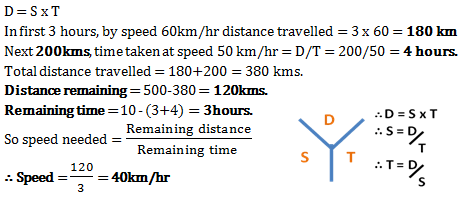# Aptitude test for MCA interview - Set 3

1)   A, B, C and D invest in a business in the ratio 3:6:2:5 respectively. But the capital was utilized in the proportion 5:3:2:6 respectively. What is share of C out of a profit of Rs. 33500?

a. Rs. 4000
b. Rs. 2000
c. Rs. 1000
d. Rs. 1500
 Answer  Explanation ANSWER: Rs. 2000 Explanation: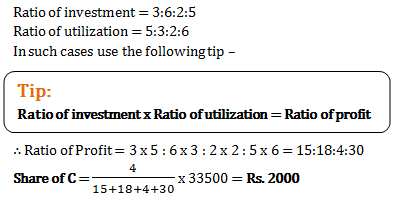2)   Ramesh was getting two offers on a book having marked price Rs. 800. Either two successive discounts of 20% each or two successive discounts of 30% and 10%. If Ramesh chose the better scheme, how much more he must have saved compared to other scheme?

a. Rs. 16
b. Rs. 24
c. Rs. 8
d. Rs. 4
 Answer  Explanation ANSWER: Rs. 16 Explanation: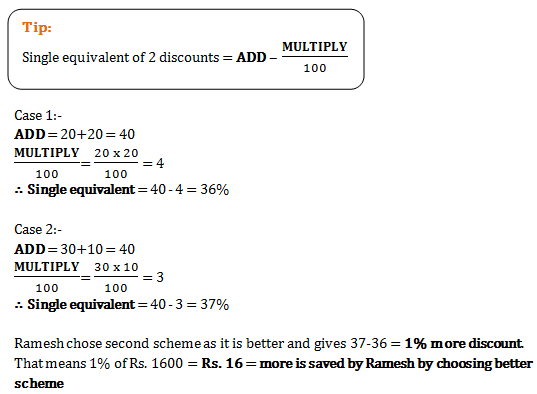3)   P, Q and R are in a cycle race of 4500 meters. P cycles twice as fast as Q. R cycles 1/3rd as fast as Q. R completes the race in 45 minutes. Then where was Q from the finishing line when P finished the race?

a. 300 m
b. 1500 m
c. 2250 m
d. 3000 m
 Answer  Explanation ANSWER: 2250 m Explanation: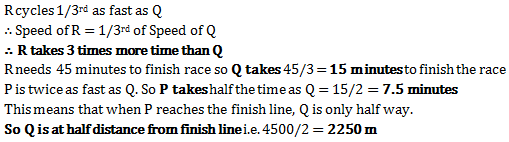4)   In Daya’s bag there are 3 books of History, 4 books of Science and 2 books of Maths. In how many ways can Daya arrange the books so that all the books of same subject are together?

a. 9
b. 6
c. 8640
d. 1728
 Answer  Explanation ANSWER: 1728 Explanation: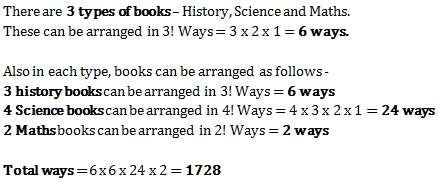5)   Ramesh’s salary was reduced by 10% and then the reduced salary was increased by 10%. What was his ultimate loss?

a. 0%
b. 10%
c. 1%
d. 5%
 Answer  Explanation ANSWER: 1% Explanation: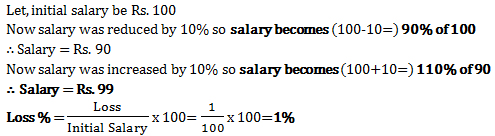6)   The price of cooking oil has increased by 25%. By what percent should a family reduce the consumption of cooking oil so as not to increase the expenditure in this account?

a. 20%
b. 25%
c. 18%
d. 16%
 Answer  Explanation ANSWER: 20% Explanation: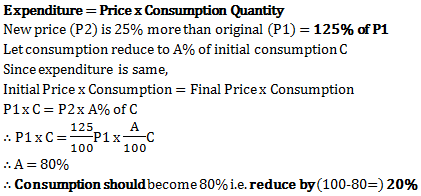7)   In a library, the ratio of number of story books to that of non-story books was 4:3 and total number of story books was 1248. When some more story books were bought, the ratio became 5:3. Find the number of story books bought.

a. 312
b. 321
c. 936
d. 1560
 Answer  Explanation ANSWER: 312 Explanation: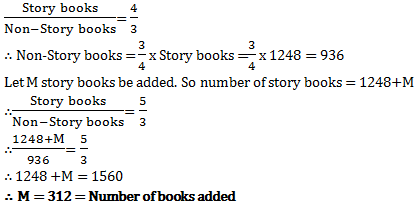8)   A sells a car to B at 10% loss. If B sells it for Rs. 54000 and gains 20%, the cost price of the car for A was

a. Rs. 25000
b. Rs. 50000
c. Rs. 37500
d. Rs. 60000
 Answer  Explanation ANSWER: Rs. 50000 Explanation: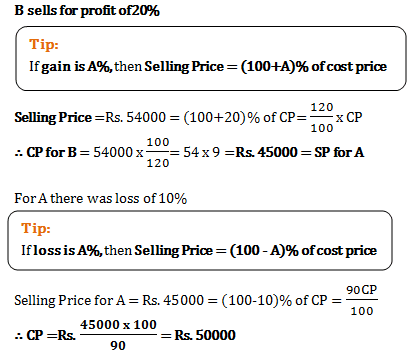9)   On a railway line there are 20 stops. A ticket is needed to travel between any 2 stops. How many different tickets would the government need to prepare to cater to all possibilities?

a. 760
b. 190
c. 380
d. 72
 Answer  Explanation ANSWER: 380 Explanation: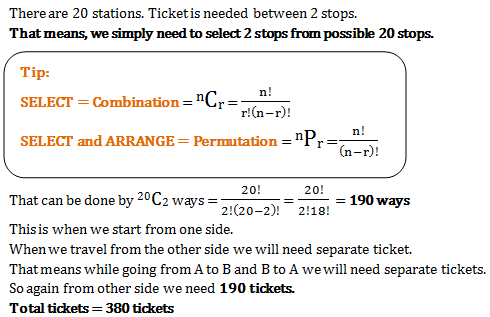10)   A’s salary is 50% more than B’s. How much percent is B’s salary less than A’s?

a. 33(1/4)%
b. 33(1/3)%
c. 33(1/2)%
d. 33%
 Answer  Explanation ANSWER: 33(1/3)% Explanation: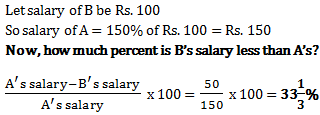11)   After deducting a commission of 5%, a TV set costs Rs. 9595. Its marked price is

a. Rs. 10000
b. Rs. 10074.75
c. Rs. 10100
d. Rs. 12000
 Answer  Explanation ANSWER: Rs. 10074.75 Explanation: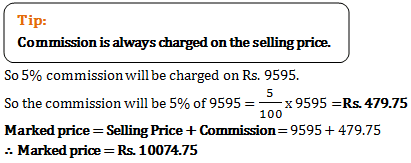12)   A fort has provision for 42 days. After 10 days, a reinforcement of 200 men arrives and the food will now last only for 24 days. How many men were there in the fort in the beginning?

a. 600
b. 575
c. 550
d. 520
 Answer  Explanation ANSWER: 600 Explanation: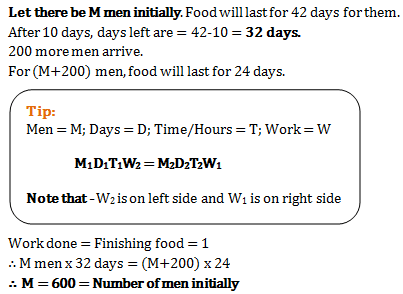13)   The ratio of the present age of father to that of son is 7:2. After 10 years their ages will be in the ratio of 9:4. The present ages of the father is

a. 35 years
b. 40 years
c. 30 years
d. 25 years
 Answer  Explanation ANSWER: 35 years Explanation: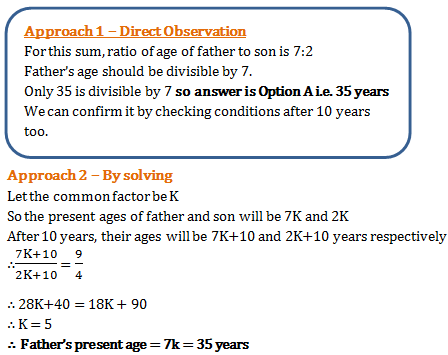14)   Rs. 8400 is divided among A, B, C and D in such a way that the shares of A and B, B and C, and C and D are in the ratios of 2:3, 4:5 and 6:7 respectively. The share of A is

a. Rs. 1280
b. Rs. 8400
c. Rs. 8210
d. Rs. 1320
 Answer  Explanation ANSWER: Rs. 1280 Explanation: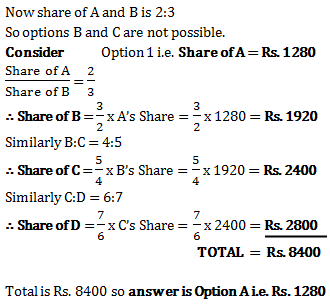15)   A fruit seller buys lemons at 2 for a rupee and sells then at 5 for three rupees. His gain percent is

a. 10%
b. 15%
c. 20%
d. 25%
 Answer  Explanation ANSWER: 20% Explanation: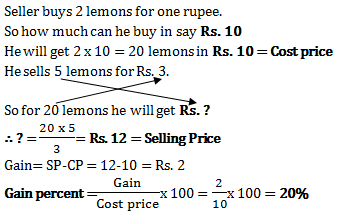16)   A person sold a stove for Rs. 423 and incurred a loss of 6%. At what price would it be sold so as to earn a profit of 8%?

a. Rs. 525
b. Rs. 500
c. Rs. 490
d. Rs. 486
 Answer  Explanation ANSWER: Rs. 486 Explanation: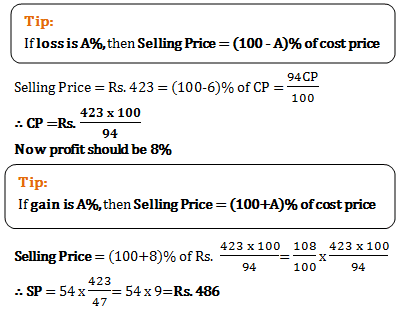17)   The surface area of a sphere is same as the curved surface area of a right circular cylinder whose height and diameter are 12cm each. The radius of the sphere is.

a. 12cm
b. 3cm
c. 6cm
d. 4cm
 Answer  Explanation ANSWER: 6cm Explanation: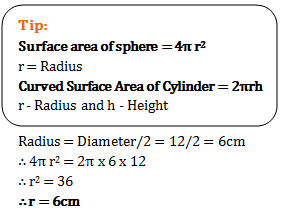18)   The value of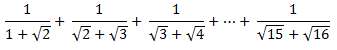a. 0
b. 3
c. -3
d. 1
 Answer  Explanation ANSWER: 3 Explanation: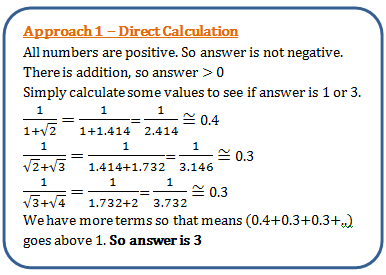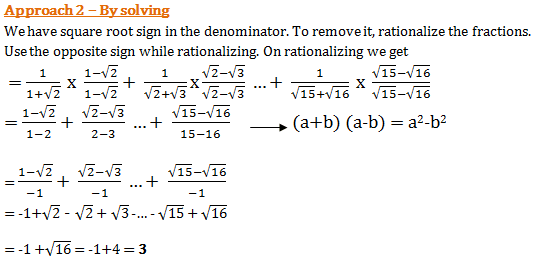19)   17 students are present in a class. In how many ways, can they be made to stand in 2 circles of 8 and 9 students?

a. 17C9 x 9! X 8!
b. 17C9 x 8! X 7!
c. 8! X 7!
d. 17C8 x 8! X 9!
 Answer  Explanation ANSWER: 17C9 x 8! X 7! Explanation: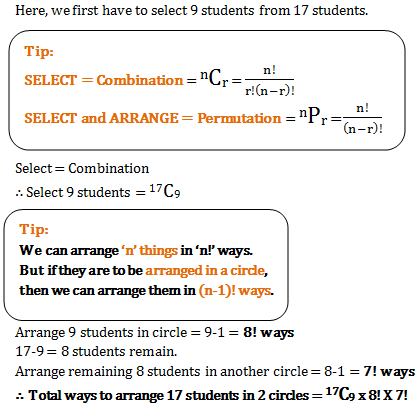20)   Rohit has to reach Mumbai which is 500 km away in 10 hours. His starting speed for 3 hours was 60 km/hr. For the next 200 km his speed was 50km/hr. By what speed he must travel now so as to reach Mumbai in decided time of 10 hours?

a. 40km/hr
b. 50 km/hr
c. 60 km/hr
d. 20 km/hr
 Answer  Explanation ANSWER: 40km/hr Explanation: